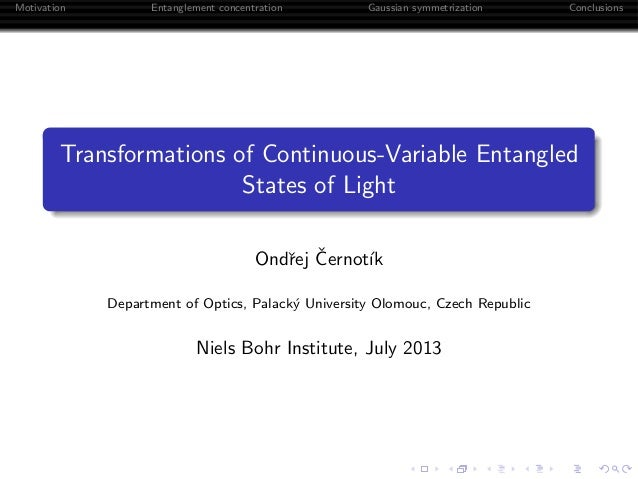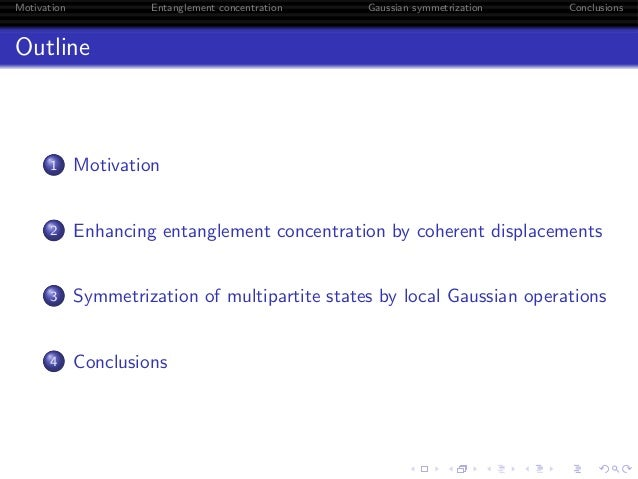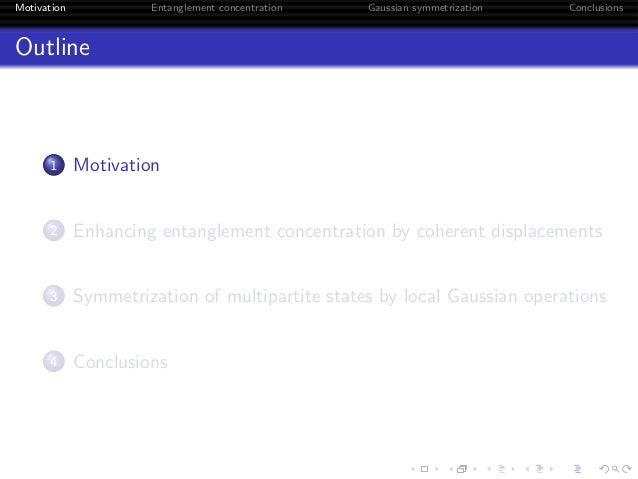Successfully reported this slideshow.Upcoming SlideShare
×

ofUpcoming SlideShare
Next

0 Likes

Share

# Transformations of continuous-variable entangled states of light

Gaussian states and Gaussian transformations represent an interesting counterpart to two-level photonic systems in the field of quantum information processing. On the theoretical side, Gaussian states are easily described using first and second moments of the quadrature operators; from the experimental point of view, Gaussian operations can be implemented using linear optics and optical parametric amplifiers. The biggest advantage compared to two-level photonic systems, is deterministic generation of entangled states in parametric amplifiers and highly efficient homodyne detection. In this presentation, we propose new protocols for manipulation of entanglement of Gaussian states.
Firstly, we study entanglement concentration of split single-mode squeezed vacuum states by photon subtraction enhanced by local coherent displacements. These states can be obtained by mixing a single-mode squeezed vacuum state with vacuum on a beam splitter and are, therefore, generated more easily than two-mode squeezed vacuum states. We show that performing local coherent displacements prior to photon subtraction can lead to an enhancement of the output entanglement. This is seen in weak-squeezing approximation where destructive quantum interference of dominant Fock states occurs, while for arbitrarily squeezed input states, we analyze a realistic scenario, including limited transmittance of tap-off beam splitters and limited efficiency of heralding detectors.
Next, motivated by results obtained for bipartite Gaussian states, we study symmetrization of multipartite Gaussian states by local Gaussian operations. Namely, we analyze strategies based on addition of correlated noise and on quantum non-demolition interaction. We use fidelity of assisted quantum teleportation as a figure of merit to characterize entanglement of the state before and after the symmetrization procedure. Analyzing the teleportation protocol and considering more general transformations of multipartite Gaussian states, we show that the fidelity can be improved significantly.

See all

### Related Audiobooks

#### Free with a 30 day trial from Scribd

See all
• Be the first to like this

### Transformations of continuous-variable entangled states of light

1. 1. Motivation Entanglement concentration Gaussian symmetrization Conclusions Transformations of Continuous-Variable Entangled States of Light Ondˇrej ˇCernot´ık Department of Optics, Palack´y University Olomouc, Czech Republic Niels Bohr Institute, July 2013
2. 2. Motivation Entanglement concentration Gaussian symmetrization Conclusions Outline 1 Motivation 2 Enhancing entanglement concentration by coherent displacements 3 Symmetrization of multipartite states by local Gaussian operations 4 Conclusions
3. 3. Motivation Entanglement concentration Gaussian symmetrization Conclusions Outline 1 Motivation 2 Enhancing entanglement concentration by coherent displacements 3 Symmetrization of multipartite states by local Gaussian operations 4 Conclusions
4. 4. Motivation Entanglement concentration Gaussian symmetrization Conclusions Gaussian States Wigner Function W (x) = 1 2π √ det γ exp − 1 2 (x − ¯x)T γ−1 (x − ¯x) Mathematical description in phase space. Feasible using linear optics, squeezers and homodyne detection.1 1 S. L. Braunstein and P. van Loock, RMP 77, 513, C. Weedbrook et al., RMP 84, 621
5. 5. Motivation Entanglement concentration Gaussian symmetrization Conclusions Quantum Entanglement Important resource in quantum information processing. Applications of Entanglement Quantum teleportation, Quantum key distribution, Quantum dense coding, One-way quantum computing, Quantum metrology,. . .
6. 6. Motivation Entanglement concentration Gaussian symmetrization Conclusions Bipartite and Multipartite Entanglement Bipartite Entanglement Relatively easy identiﬁcation and quantiﬁcation.
7. 7. Motivation Entanglement concentration Gaussian symmetrization Conclusions Bipartite and Multipartite Entanglement Bipartite Entanglement Relatively easy identiﬁcation and quantiﬁcation. Multipartite Entanglement Complexity grows with number of parties. (Tripartite Gaussian entanglement – 5 entanglement classesa). Problematic quantiﬁcation. Applications: one-way quantum computingb, quantum networksc. a G. Giedke et al., PRA 64, 052303 b N. C. Menicucci et al., PRL 97, 110501 c P. van Loock and S. L. Braunstein, PRL 84, 3482
8. 8. Motivation Entanglement concentration Gaussian symmetrization Conclusions Outline 1 Motivation 2 Enhancing entanglement concentration by coherent displacements 3 Symmetrization of multipartite states by local Gaussian operations 4 Conclusions
9. 9. Motivation Entanglement concentration Gaussian symmetrization Conclusions Why Entanglement Concentration? Distribution of entangled states is subject to losses and decoherence. State degradation can be probabilistically eliminated using local operations and classical communication.
10. 10. Motivation Entanglement concentration Gaussian symmetrization Conclusions Entanglement Concentration and CV Systems Non-Gaussianity Required Gaussian states → non-Gaussian operations.a Non-Gaussian states → Gaussian operations.b a J. Eisert et al., PRL 89, 137903, J. Fiur´aˇsek, PRL 89, 137904 b R. Dong et al., Nat. Phys. 4, 919, B. Haage et al., Nat. Phys. 4, 915
11. 11. Motivation Entanglement concentration Gaussian symmetrization Conclusions Photon Subtraction Unbalanced beam splitter and single-photon detection.1 Can be improved by local Gaussian operations.2 BS APD 1 H. Takahashi et al., Nat. Photon. 4, 178 2 J. Fiur´aˇsek, PRA 84, 012335, S. L. Zhang and P. van Loock, PRA 84, 062309
12. 12. Motivation Entanglement concentration Gaussian symmetrization Conclusions The Protocol ˆD(α) ˆD(−α) ˆF = ˆa + α
13. 13. Motivation Entanglement concentration Gaussian symmetrization Conclusions The Protocol ˆD(α) ˆD(−α) ˆF = ˆa + α ˆa + α ˆF1 = ˆa + α
14. 14. Motivation Entanglement concentration Gaussian symmetrization Conclusions The Protocol ˆD(α) ˆD(−α) ˆF = ˆa + α ˆa + α ˆF1 = ˆa + α ˆa + α ˆb + β ˆF2 = (ˆa + α) ⊗ (ˆb + β)
15. 15. Motivation Entanglement concentration Gaussian symmetrization Conclusions Input State Single-mode squeezed vacuum split on a beam splitter. |ψin = 4 1 − λ2 ∞ n=0 2n k=0 λn 2nn! (2n)!t2n−krk k!(2n − k)! |2n − k, k
16. 16. Motivation Entanglement concentration Gaussian symmetrization Conclusions Weak Input Squeezing Zero- and two-photon contributions, |ψin ≈ |00 + λrt|11 + λ √ 2 (t2 |20 + r2 |02 ). Destructive quantum interference leads to enhancement of entanglement.
17. 17. Motivation Entanglement concentration Gaussian symmetrization Conclusions Weak Input Squeezing Single-Mode Subtraction |ψ1 = λt(t|10 + r|01 ) + α|ψin Zero displacement is optimal. 0.0 0.2 0.4 0.6 0.8 1.0 E -0.05 0.0 0.05
18. 18. Motivation Entanglement concentration Gaussian symmetrization Conclusions Weak Input Squeezing Two-Mode Subtraction |ψ2 = (λrt + αβ)|00 + λ(αr + βt)(t|10 + r|01 ) + + λ √ 2 αβ(t2 |20 + √ 2rt|11 + r2 |02 ) -0.15 0.0 0.15 -0.15 0.0 0.15
19. 19. Motivation Entanglement concentration Gaussian symmetrization Conclusions Weak Input Squeezing Two-Mode Subtraction |ψ2 = (λrt + αβ)|00 + λ(αr + βt)(t|10 + r|01 ) + + λ √ 2 αβ(t2 |20 + √ 2rt|11 + r2 |02 ) Vacuum term elimination, αβ = −λrt. |ψ2 = √ 2rt|11 +t2|20 +r2|02 -0.15 0.0 0.15 -0.15 0.0 0.15
20. 20. Motivation Entanglement concentration Gaussian symmetrization Conclusions Weak Input Squeezing Two-Mode Subtraction |ψ2 = (λrt + αβ)|00 + λ(αr + βt)(t|10 + r|01 ) + + λ √ 2 αβ(t2 |20 + √ 2rt|11 + r2 |02 ) Vacuum term elimination, αβ = −λrt. Single-photon contributions, α = √ λt, β = √ λr. |ψ2 = √ 2rt|11 +t2|20 +r2|02 0.8 1.0 1.2 1.4 1.6 E 0.0 0.05 0.1 0.15
21. 21. Motivation Entanglement concentration Gaussian symmetrization Conclusions Arbitrary Squeezing A Realistic Scenario Experimental Realization Stronger squeezing. On-oﬀ detectors for photon subtraction with limited eﬃciency. Finite transmittance of tap-oﬀ beam splitters.
22. 22. Motivation Entanglement concentration Gaussian symmetrization Conclusions Arbitrary Squeezing A Realistic Scenario Experimental Realization Stronger squeezing. On-oﬀ detectors for photon subtraction with limited eﬃciency. Finite transmittance of tap-oﬀ beam splitters. Higher photon numbers, mixed output state, more complicated ﬁltering operation.
23. 23. Motivation Entanglement concentration Gaussian symmetrization Conclusions Arbitrary Squeezing Results 0.0 0.2 0.4 0.6 0.8 1.0 1.2 EN -0.5 0.0 0.5 0.9 1.0 1.1 1.2 EN 0.0 0.1 0.2 0.3 0.4 10 -7 10 -6 10 -5 10 -4 10 -3 10 -2 P1 0.0 0.1 0.2 0.3 0.4 -1.0 -0.5 0.0 0.5 1.0 -1.0 -0.5 0.0 0.5 1.0 1.3 1.4 1.5 EN 0.0 0.1 0.2 0.3 0.4 10 -14 10 -12 10 -10 10 -8 10 -6 10 -4 10 -2 P2 0.0 0.1 0.2 0.3 0.4
24. 24. Motivation Entanglement concentration Gaussian symmetrization Conclusions Arbitrary Squeezing Results Conclusions Single-mode subtraction optimal without displacements. Two-mode subtraction gives more output entanglement; the success probability is smaller. a O. ˇCernot´ık and J. Fiur´aˇsek, PRA 86, 052339
25. 25. Motivation Entanglement concentration Gaussian symmetrization Conclusions Arbitrary Squeezing Results Conclusions Single-mode subtraction optimal without displacements. Two-mode subtraction gives more output entanglement; the success probability is smaller. a O. ˇCernot´ık and J. Fiur´aˇsek, PRA 86, 052339 Extensions Losses limit usability of the protocol. No Gaussian entanglement at the output. b A. Tipsmark et al., Opt. Exp. 21, 6670
26. 26. Motivation Entanglement concentration Gaussian symmetrization Conclusions Outline 1 Motivation 2 Enhancing entanglement concentration by coherent displacements 3 Symmetrization of multipartite states by local Gaussian operations 4 Conclusions
27. 27. Motivation Entanglement concentration Gaussian symmetrization Conclusions Symmetrization of Multipartite Gaussian States Equalization of quadrature correlations.         n 0 c 0 c 0 0 n 0 −d 0 −d c 0 n 0 c 0 0 −d 0 n 0 −d c 0 c 0 n 0 0 −d 0 −d 0 n         →         n′ 0 c′ 0 c′ 0 0 n′ 0 −c′ 0 −c′ c′ 0 n′ 0 c′ 0 0 −c′ 0 n′ 0 −c′ c′ 0 c′ 0 n′ 0 0 −c′ 0 −c′ 0 n′         Generalization of protocols for bipartite Gaussian states.1 1 J. Fiur´aˇsek, PRA 86, 032317
28. 28. Motivation Entanglement concentration Gaussian symmetrization Conclusions Equivalent State Preparation N 1 2 . . . rN , nN 1:12:1(N − 1):1 r1, n1 r1, n1 r1, n1r1, n1 BS1 BSN−2 BSN−1BS2 (N − 2):1 N − 2 N − 1 Simpliﬁed analysis – working with two separable modes. Similarity to experimental realizations of quantum networks.1 1 T. Aoki et al., PRL 91, 080404, H. Yonezawa et al., Nature 431, 430
29. 29. Motivation Entanglement concentration Gaussian symmetrization Conclusions Equivalent State Preparation N 1 2 . . . rN , nN 1:12:1(N − 1):1 r1, n1 r1, n1 r1, n1r1, n1 BS1 BSN−2 BSN−1BS2 (N − 2):1 N − 2 N − 1 n = 1 N [nNe2rN + (N − 1)n1e2r1 ] c = 1 N (nNe2rn − n1e2r1 ) d = 1 N (n1e−2r1 − nNe−2rN )
30. 30. Motivation Entanglement concentration Gaussian symmetrization Conclusions Assisted Quantum Teleportation Entanglement characterization – assisted teleportation ﬁdelity.1 F = 1√ (n−c+1)(n−d+1−2d2/n) More general transformations (n, c, d) → (n′, c′, kc′). A B C in (qin − qA)/ √ 2 (pin + pA)/ √ 2 1 P. van Loock and S.L. Braunstein, PRL 84, 3482
31. 31. Motivation Entanglement concentration Gaussian symmetrization Conclusions Strategies Correlated Noise Addition ργNS γN S γN S Adding correlated noise γN. Squeezing S.
32. 32. Motivation Entanglement concentration Gaussian symmetrization Conclusions Strategies Quantum Non-Demolition Interaction ρgdAS g dB S g dC S QND interaction g, measurement on ancillas and displacement d. Squeezing S.
33. 33. Motivation Entanglement concentration Gaussian symmetrization Conclusions Results for Tripartite States Noise Addition 0.45 0.5 0.55 0.6 0.65 F 0.45 0.5 0.55 0.6 0.65 0.7 0.75 k QND Interaction 0.4 0.45 0.5 0.55 F 0.3 0.4 0.5 0.6 0.7 k Both strategies work best for noisy states.
34. 34. Motivation Entanglement concentration Gaussian symmetrization Conclusions Outlook More general class of transformations, (n1, n2, c, d) → (n′, k1n′, c′, k2c′). Formalism of complex symplectic matrices for purity-preserving Gaussian quantum ﬁlters.1 1 J. Fiur´aˇsek, PRA 87, 052301
35. 35. Motivation Entanglement concentration Gaussian symmetrization Conclusions Outline 1 Motivation 2 Enhancing entanglement concentration by coherent displacements 3 Symmetrization of multipartite states by local Gaussian operations 4 Conclusions
36. 36. Motivation Entanglement concentration Gaussian symmetrization Conclusions Entanglement Concentration Value of Squeezing Weak squeezing: Destructive quantum interference. Arbitrary squeezing: Realistic experimental scenario.
37. 37. Motivation Entanglement concentration Gaussian symmetrization Conclusions Entanglement Concentration Value of Squeezing Weak squeezing: Destructive quantum interference. Arbitrary squeezing: Realistic experimental scenario. Strategies Single-mode photon subtraction optimal without displacements. Local displacements can improve two-mode subtraction.
38. 38. Motivation Entanglement concentration Gaussian symmetrization Conclusions Entanglement Concentration Value of Squeezing Weak squeezing: Destructive quantum interference. Arbitrary squeezing: Realistic experimental scenario. Strategies Single-mode photon subtraction optimal without displacements. Local displacements can improve two-mode subtraction. Structure of the entanglement.
39. 39. Motivation Entanglement concentration Gaussian symmetrization Conclusions Symmetrization of multipartite Gaussian states Tools Equivalent state preparation for analyzing protocols. Assisted teleportation ﬁdelity for state characterization.
40. 40. Motivation Entanglement concentration Gaussian symmetrization Conclusions Symmetrization of multipartite Gaussian states Tools Equivalent state preparation for analyzing protocols. Assisted teleportation ﬁdelity for state characterization. Strategies Correlated noise addition: More sensitive to imperfections (narrow peak). QND interaction: More challenging experimentally (use of atomic ensemblesa, linear optical emulationb). Each strategy optimal for diﬀerent types of states. a K. Hammerer et al., RMP 82, 1041 b R. Filip et al., PRA 71, 042308
41. 41. Motivation Entanglement concentration Gaussian symmetrization Conclusions Symmetrization of multipartite Gaussian states Tools Equivalent state preparation for analyzing protocols. Assisted teleportation ﬁdelity for state characterization. Strategies Correlated noise addition: More sensitive to imperfections (narrow peak). QND interaction: More challenging experimentally (use of atomic ensemblesa, linear optical emulationb). Each strategy optimal for diﬀerent types of states. a K. Hammerer et al., RMP 82, 1041 b R. Filip et al., PRA 71, 042308 Possible extensions of the protocol.
42. 42. Motivation Entanglement concentration Gaussian symmetrization Conclusions Credits Jarom´ır Fiur´aˇsek Radim Filip Financial support: Thank you for your attention!

Total views

66

On Slideshare

0

From embeds

0

Number of embeds

0

2

Shares

0